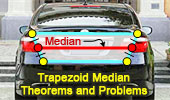# Median of a Trapezoid, Theorems and Problems- Table of ContentTrapezoids. Index.

Proposed Problem 237. Quadrilateral, Midpoints, Exterior line, Perpendicular lines.
Median of a Trapezoid.

Proposed Problem 236. Quadrilateral, Midpoints, Exterior line, Perpendicular lines.
Median of a Trapezoid.

Proposed Problem 231. Triangle, Midpoints, Transversal, Perpendicular lines.
Median of a Trapezoid.

Proposed Problem 230. Triangle, Midpoints, Transversal, Perpendicular lines.

Proposed Problem 229. Triangle, Centroid, Transversal, Perpendicular lines.
Median of a Trapezoid.

Proposed Problem 228. Triangle, Midpoints, Exterior line, Perpendiculars lines.
Median of a Trapezoid.

Proposed Problem 227. Triangle, Centroid, Exterior line, Perpendicular lines.
Median of a Trapezoid.

Proposed Problem 226. Triangle, Centroid, Perpendiculars.
Median of a Trapezoid.

Proposed Problem 219. Rhombus, perpendiculars, arithmetic mean.
Midpoints. Median of a Trapezoid.

Proposed Problem 218. Right triangle, Altitude and Projections.
Midpoints. Median of a Trapezoid.

Proposed Problem 217. Right triangle, Altitude and Projections.
Midpoints. Median of a Trapezoid.

Trapezoid, Triangle, Diagonals, Midpoints. Dynamic Geometry.
Step-by-Step construction, Manipulation, and animation.
Prove proposition.

Proposed Problem 170. Trapezoid, Midpoint, Triangle, Area. Median of a Trapezoid.

Proposed Problem 158. Relation between the Circumradius, Inradius and Exradii of a triangle. Median of a Trapezoid.

Trapezoid

TRAPEZOID: A trapezoid is a quadrilateral having two parallel sides called bases.SCALENE, ISOSCELES, AND RIGHT TRAPEZOID:MEDIAN OF A TRAPEZOID (Midline or Midsegment):  Segment joining the midpoints of the two legs. The median is parallel to the bases and equal to one half of the sum of the bases.MIDPOINTS OF THE DIAGONALS:  The segment joining the midpoints of the two diagonals is parallel to the bases and equal to one half of the difference of the bases.Home | Search | Geometry | Quadrilateral | Trapezoid | Email | By Antonio Gutierrez